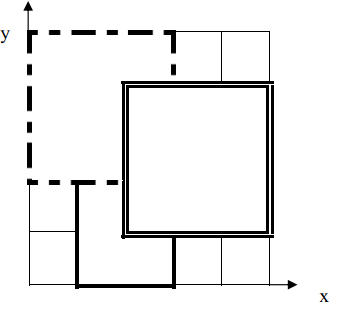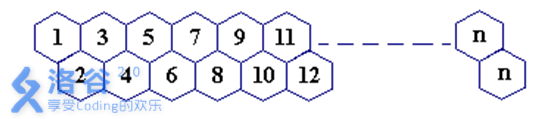# 2022-3-21（洛谷）

### 铺地毯（模拟+枚举）

3
1 0 2 3
0 2 3 3
2 1 3 3
2 2


3


3
1 0 2 3
0 2 3 3
2 1 3 3
4 5

-1

【样例解释 1】【数据范围】

#include<iostream>
using namespace std;

//铺地毯
int n,a,b,g,k,id;
int x,y;
int main()
{
cin>>n;
for(int i=1;i<=n;i++){
id[i]=i;//地毯编号
scanf("%d%d%d%d",&a[i],&b[i],&g[i],&k[i]);
}
scanf("%d%d",&x,&y);
int f=0,index;
for(int i=n;i>=1;i--){//逆序寻找
if(x>=a[i]&&x<=a[i]+g[i]&&y>=b[i]&&y<=b[i]+k[i]){
f=1;
index=id[i];
break;
}
}
if(f) cout<<index<<endl;
else cout<<-1<<endl;
return 0;
}

### 独木桥（模拟+思维）

4
2
1 3


2 4

#include<iostream>
using namespace std;

//独木桥
int L,N,x;//桥长，士兵数，初始坐标
int maxt=0,mint=0;//最长，最短 时间
int main()
{
scanf("%d%d",&L,&N);
for(int i=1;i<=N;i++){
scanf("%d",&x);
mint=max(mint,min(x,L-x+1));
maxt=max(maxt,max(x,L-x+1));
}
cout<<mint<<" "<<maxt<<endl;
return 0;
}

### 数楼梯（高精+斐波那契数列）

4

5

• 对于 60% 的数据，0N≤50；// 用long long即可
• 对于 100% 的数据，1≤N≤5000//高精度

n=1,f(1)=1; (1)

n=2,f(2)=2; (1 1, 2)

n=3,f(3)=3; (1 1 1, 1 2 , 2 1)

n=4,f(4)=5; (1 1 1 1, 2 2, 1 1 2, 1 2 1, 2 1 1)

n=5,f(5)=8; (1 1 1 1 1, 1 1 1 2, 1 1 2 1, 1 2 1 1 , 2 1 1 1, 2 2 1, 1 2 1, 1 2 2)

... ...

#include<iostream>
using namespace std;

//数楼梯(高精斐波那契数列)
int f,n,len;//f[i][j]:第i阶台阶对应的走法数
void fun(int k){//高精加
for(int i=1;i<=len;i++)
f[k][i]=f[k-1][i]+f[k-2][i];
for(int i=1;i<=len;i++){//进位
if(f[k][i]>=10){
f[k][i+1]+=f[k][i]/10;
f[k][i]%=10;
if(f[k][len+1]>0) len++;
}
}
}
int main()
{
cin>>n;
len=1;
f=1,f=2;//初始化
for(int i=3;i<=n;i++)
fun(i);
for(int i=len;i>=1;i--) cout<<f[n][i];
return 0;
}

### 蜜蜂路线（与上题一样一样的）1 14

377

#include<iostream>
using namespace std;

//蜜蜂路线
int n,m,f,len;
void fun(int k){
for(int i=1;i<=len;i++)
f[k][i]=f[k-1][i]+f[k-2][i];//每一位分别用公式计算
for(int i=1;i<=len;i++){
if(f[k][i]>=10){
f[k][i+1]+=f[k][i]/10;
f[k][i]%=10;
}
if(f[k][len+1]>0) len++;//如果进位了，下次需计算len++次
}
}
int main()
{
cin>>m>>n;
len=1;
f=1,f=2;
for(int i=3;i<=n-m;i++)
fun(i);
for(int i=len;i>=1;i--) cout<<f[n-m][i];//递推或者列举几个找到规律
return 0;
}


### 数的计算(递推)

1. 不作任何处理；

2. 在它的左边拼接一个正整数，但该正整数不能超过原数的一半；

3. 加上数后，继续按此规则进行处理，直到不能再加正整数为止。

6


6

【样例解释】满足条件的数为：6，16，26，126，36，136。


f(1)=1; // 1
f(2)=f(2/2)+1; // 1, 12
f(3)=f(3/2)+1; // 1, 13
f(4)=f(1)+f(4/2)+1; // 1, 14, 24, 124
f(5)=f(1)+f(5/2)+1; // 1, 15, 25, 125
f(6)=f(1)+f(2)+f(6/2)+1;
... ...
f(n)=f(1)+f(2)+...+f(n/2)+1;
#include<iostream>
using namespace std;

//数的计算
int n,f;
int main()
{
cin>>n;
for(int i=1;i<=n;i++){
for(int j=1;j<=i/2;j++){
f[i]+=f[j];
}
f[i]++;//最后加上自己
}
cout<<f[n]<<endl;
return 0;
}
08-10
05-204498
08-233309
09-252万+
10-092678
06-284481
04-181222
08-281110
09-21
07-14
01-01
10-31
10-088126
12-02274
05-25

### “相关推荐”对你有帮助么？

•非常没帮助
•没帮助
•一般
•有帮助
•非常有帮助被折叠的  条评论 为什么被折叠?到【灌水乐园】发言-小透明-

¥2 ¥4 ¥6 ¥10 ¥20余额支付 (余额：-- )扫码支付获取中扫码支付点击重新获取扫码支付1.余额是钱包充值的虚拟货币，按照1:1的比例进行支付金额的抵扣。
2.余额无法直接购买下载，可以购买VIP、C币套餐、付费专栏及课程。余额充值